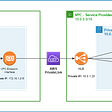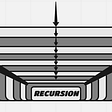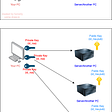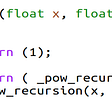# Remove Nth Node From End of List — Day 107(Python)

Today we will be working on the LinkedList problem. Let’s look into the problem statement.

19. Remove Nth Node From End of List

Given the `head` of a linked list, remove the `nth` node from the end of the list and return its head.

Example 1:

`Input: head = [1,2,3,4,5], n = 2Output: [1,2,3,5]`

Example 2:

`Input: head = , n = 1Output: []`

Example 3:

`Input: head = [1,2], n = 1Output: `

Constraints:

• The number of nodes in the list is `sz`.
• `1 <= sz <= 30`
• `0 <= Node.val <= 100`
• `1 <= n <= sz`

Follow up: Could you do this in one pass?

One way to solve this problem is to find at what position we want to remove the node from. To identify that position, first, find the length of the linked list, then subtract N from the length of the linked list.

After finding the position, we will traverse through the linked list and then delete the node in that position. We will use 3 variables that point to the previous node, current node, and next node. Once the current node reaches the required position, we will point the previous node to the next node, thus removing the current node.

Let us look into the code.

`# Definition for singly-linked list.# class ListNode:#     def __init__(self, val=0, next=None):#         self.val = val#         self.next = nextclass Solution:    def length_ll(self, head):        length_of_linkedlist = 0        while(head):            length_of_linkedlist += 1            head = head.next        return length_of_linkedlist        def removeNthFromEnd(self, head: Optional[ListNode], n: int) -> Optional[ListNode]:        length_of_linkedlist = self.length_ll(head)        prev, curr = None, head        position_to_remove = length_of_linkedlist - n        if(length_of_linkedlist == 1 and n == 1 or position_to_remove == 0):            return head.next        while(curr):            nex = curr.next            if(position_to_remove == 0):                prev.next = nex                break            else:                prev = curr                curr = nex                position_to_remove -= 1        return head`

Complexity Analysis

Time Complexity

We are traversing through the linked list twice; once to find the length of the linked list and second to remove the node. The time complexity is O(N) where N is the length of the Linked list.

Space Complexity

We are not using any extra space and hence space complexity is O(1).

How can we solve this problem in one pass? I had to look into the solution to understand that having two-pointers are considered as one pass.

Pointer 1 will move until we reach n. Once pointer 1 reaches n, we will start pointer 2 until pointer 1 reaches the end of the linked list. The node which pointer 2 points at, is the node that needs to be removed.

`# Definition for singly-linked list.# class ListNode:#     def __init__(self, val=0, next=None):#         self.val = val#         self.next = nextclass Solution:    def removeNthFromEnd(self, head: Optional[ListNode], n: int) -> Optional[ListNode]:        if head.next is None:            return None        fast,slow=head,head        for i in range(n):            fast=fast.next        if not fast:            return head.next        while fast.next:            slow=slow.next            fast=fast.next        if n==1:            slow.next=None        else:            slow.next=slow.next.next        return head`

Complexity Analysis

Time Complexity

We are traversing through the linked list using two pointers, hence time complexity is O(N).

Space Complexity

We are not using any extra space and hence space complexity is O(1).

--

--

--

## More from Annamariya Tharayil

Software Engineer. Find me @ www.linkedin.com/in/annamariya-jt

Love podcasts or audiobooks? Learn on the go with our new app.

## Restarting HTTPD Service is not idempotence in nature and also consume more resources suggest a way…## Brass Golem Beta 0.22.1## How to Securely Connect to Provider’s service via AWS PrivateLink## Image Processing using OpenCV## How do I DevOpsify all that as-built stuff?## 10 Essential Data Science Packages for Python## Deploying environment variables for Firebase Cloud Functions## Migrate API: custom Drupal-to-Drupal migration## Annamariya Tharayil

Software Engineer. Find me @ www.linkedin.com/in/annamariya-jt

## Recursion and stack## How to get into a Remote Server — Part 2 & 3## Understanding Recursion and Stack## Number of Good Pairs — Day 101(Python)